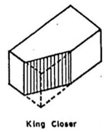Engineering Jobs   »   Civil Engineering Quiz

# RSMSSB-JE’21 CE: Daily Practices Quiz. 23-July-2021

Quiz: Civil Engineering
Exam: RSMSSB-JEn
Topic: Miscellaneous

Each question carries 1 mark
Negative marking: 1/3 mark
Time: 8 Minutes

Q1. The true length of a line is known to be 200 m. When this is measured with a 20 m tape, the length is 200.80 m.
What is the correct length of the 20 m tape?
(a) 19.98 m
(b) 20.08 m
(c) 20.04 m
(d) 19.92 m

Q2. Calculate the capitalized value of a building having annual rent of Rs. 20,000 and highest rate of interest is 5%
(a) 1000
(b) 21000
(c) 220000
(d) 400000

Q3. The portion of the brick without a triangular corner equal to half the width and half the length is called ………..
(a) Closer
(b) Queen closer
(c) King closer
(d) Squint brick

Q4. According to IS 399-1963, the weight of the timber is to be recommended at a moisture content of ________.
(a) 4%
(b) 12%
(c) 8%
(d) 5%

Q5. For a given soil sample,
C_c= Coefficient of curvature
C_u = Coefficient of uniformity
D_10 = Effective size
D_30= Diameter through which 30% of the total soil mass is passing
If C_c=1.0 and C_u=4.0, then what would be the value of D_30 \/D_10 ?
(a) 2.00
(b) 1.25
(c) 1.50
(d) 1.75

Q6. The moment of inertia of a hollow circular section whose external diameter is 8 cm and internal diameter is 6 cm about centroidal axis is _______
(a) 437.5 cm^4
(b) 337.5 cm^4
(c) 237.5 cm^4
(d) 137.5 cm^4

Solutions

S1. Ans.(d)
Sol. Given, True length of line (L) = 200 m.
True length of Tape (l) = 20 m.
Wrong length of line (L’) = 200.80m.
Actual length of Tape (l’) =?
L × l = L’ × l’
200 × 20 = 200.8 × l’
▭(l^’=19.92 m.)

S2. Ans.(d)
Sol. Annual rent (R) = 20,000
Year purchase (Y.P) = 100/(i%)
= 100/5
▭(Y.P=20)
Capitalized value = Annual rent × Year’s Purchase
= 20,000 × 20
= 400,000 Rs.

S3. Ans.(c)
Sol. The portion of the brick without a triangular corner equal to half the width and half the length is called king closer.S4. Ans.(b)
Sol. Seasoning is the process of adjusting the moisture content of wood or remove the sap from the wood. The moisture content in a well-seasoned timber is about 10-12%
Objective of seasoning →
– Reduce the weight
– Reduction in Shrinkage and warping.
– Increase in strength and durability.
– Remove sap.
– Reduce the tendency of split and decay.

S5 Ans.(a)
Sol. C_c=1 C_u=4
(D_30 )^2/(D_60 D_10 )=1–(1) D_60/D_10 =4–(2)
Multiply both eq^n
(D_30/D_10 )^2=4 ⇒ ▭(D_30/D_10 =2)

S6. Ans.(d)
Sol. Moment of inertia of follow circular section
I=π/64 ( d_O^4-di_O^4 )
=π/64 (8^4-6^4 )
=137.44 cm^4

Sharing is caring!